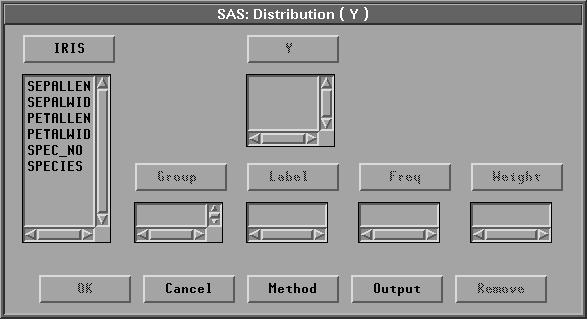Distribution Analyses

# Variables

To create a distribution analysis, choose Analyze:Distribution ( Y ). If you have already selected one or more variables, a distribution analysis for each selected variable appears. If you have not selected any variables, a variables dialog appears.Figure 38.2: Distribution Variables Dialog

Select at least one Y variable for each distribution analysis.

You can select one or more Group variables if you have grouped data. This creates one distribution analysis for each group.

You can select a Label variable to label observations in the plots.

You can select a Freq variable. If you select a Freq variable, each observation is assumed to represent n observations, where n is the value of the Freq variable.

You can select a Weight variable to specify relative weights for each observation in the analysis. The details of weighted analyses are explained in the individual sections of this chapter.